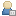Show Posts

This section allows you to view all posts made by this member. Note that you can only see posts made in areas you currently have access to.

Messages - Qedo

Pages: 1 ... 20 21  23 24
316
GLBasic - en / Re: 2D Physics Library
« on: 2012-Feb-26 »
Insert the  attached box2d files in the pogram's folder
Ciao

317
GLBasic - en / Re: 2D Physics Library
« on: 2012-Feb-26 »
I attach an example with sprites that I hope will be useful
Ciao

318
GLBasic - en / Re: array index
« on: 2012-Feb-25 »
Here the 2 methods, static and dymanic array, and benchmark

INLINE
int height4d_C = array4dLen_C; //27
int depth4d_C = array4dLen_C * array4dLen_C; //729
int time4d_C = array4dLen_C * array4dLen_C * array4dLen_C; //19683
const int array4dLen_C=27;
const int array1dLen_C=array4dLen_C * array4dLen_C * array4dLen_C * array4dLen_C;

// Fill STATIC STACK 1d array simulating 4d
static int array1d_C[array1dLen_C];
for (tc = 0; tc < array4dLen_C; tc++)
for (zc = 0; zc < array4dLen_C; zc++)
for (yc = 0; yc < array4dLen_C; yc++)
for (xc = 0; xc < array4dLen_C; xc++)
array1d_C[xc + yc * height4d_C + zc * depth4d_C + tc * time4d_C] = i;

// Fill DYNAMIC HEAP 1d array simulating 4d
int *array4dd_C;
array4dd_C = new int[array4dLen_C * array4dLen_C * array4dLen_C * array4dLen_C];
for (tc = 0; tc < array4dLen_C; tc++)
for (zc = 0; zc < array4dLen_C; zc++)
for (yc = 0; yc < array4dLen_C; yc++)
for (xc = 0; xc < array4dLen_C; xc++)
array4dd_C[xc + yc * height4d_C + zc * depth4d_C + tc * time4d_C] = i;
delete[] array4dd_C;
ENDINLINE

Time comparation for 531441 int array
STATIC STACK ARRAY= 0,6 seconds
DYNAMIC HEAP ARRAY= 1,8 seconds

Ciao

319
GLBasic - en / Re: array index
« on: 2012-Feb-15 »
Thank you hardyx
now work!!!Ciao

INLINE
int tc, zc, yc;
const int array3dLen_C=81;
static int array3d_C[array3dLen_C][array3dLen_C][array3dLen_C];
for (tc = 0; tc < array3dLen_C; tc++)
for (zc = 0; zc < array3dLen_C; zc++)
for (yc = 0; yc < array3dLen_C; yc++)
array3d_C[yc][zc][tc] = i;
ENDINLINE

320
GLBasic - en / array index
« on: 2012-Feb-12 »
hello all.
where is the mistake?
with  array3dLen_C = 80 all OK. With upper indices there is the error:
EXCEPTION_STACK_OVERFLOW.

ciao

INLINE
int tc, zc, yc;
int array3dLen_C=80;
int array3d_C[array3dLen_C][array3dLen_C][array3dLen_C];
for (tc = 0; tc < array3dLen_C; tc++)
for (zc = 0; zc < array3dLen_C; zc++)
for (yc = 0; yc < array3dLen_C; yc++)
array3d_C[yc][zc][tc] = i;
ENDINLINE

321
GLBasic - en / Re: Arrays in Types?
« on: 2011-Nov-13 »
Try:

CONSTANT MaxDims=3
TYPE Point
Loc[]
ENDTYPE
LOCAL P1 AS Point
REDIM P1.Loc[MaxDims]
FOR i = 0 TO MaxDims-1
IF P1.Loc=0 THEN P1.Loc=10
NEXT

Ciao

322
Inline / 3rd party / Re: return a C/C++ array to GLBasic (INLINE)
« on: 2011-Sep-28 »
It may seem obvious but it is true. Many thanks for informations and availability
Ciao

323
Code Snippets / Re: POW speed
« on: 2011-Sep-18 »
But where it is very useful in calculations of the type Bezier algorithm:
B(t)=(1-t)5P1 + 5t(1-t)4P2 + 10t2(1-t)3P3 + 10t3(1-t)2P4 + 5t4(1-t)P5+ t5P6
Ciao

324
Code Snippets / Re: POW speed
« on: 2011-Sep-18 »
I forgot to add that the elevations should not be decimal.
3, 5, 6, -4, -6 yes
-3,5, 5.67   no
Ciao

325
Code Snippets / Re: POW speed
« on: 2011-Sep-18 »
Right
Try this for negative elevations (not too complicated).   Is always faster
Obviously this program does not work for elevations greater than 0 and less than 1
Ciao

326
Code Snippets / POW speed
« on: 2011-Sep-17 »
Don't use POW function  for value of power less or ugual to 40. It is very slow
Try this code
Ciao

327
Inline / 3rd party / Re: return a C/C++ array to GLBasic (INLINE)
« on: 2011-Sep-05 »
Thank you very much.
Excuse for the obvious question, but for me is not trivial the difference between
const char*** pArrayptr
and
DGInt** pArrayptr
I'm going to studyCiao

328
Inline / 3rd party / Re: return a C/C++ array to GLBasic (INLINE)
« on: 2011-Sep-03 »
Good example but it is possible have a example with integer and float array?
Thank you
Ciao

329
GLBasic - en / float matrix multiplication benchmark
« on: 2011-Aug-29 »
I ask two things to those more experienced than me.
1) between GLB and C, in the float matrix multiplication benchmark, why there is a relationship in the rate of 5/1?   1400ms GLBasic and 300ms INLINE C. I think too high
2) Why the local arrays slow the program by 60%? LOCAL=2300ms against GLOBAL=1400ms.
To see this uncomment:
// LOCAL  a[]
// LOCAL  b[]
// LOCAL  c[]
Ciao